Скачать презентацию CVPR 10 Common Visual Pattern Discovery via Spatially

• Количество слайдов: 24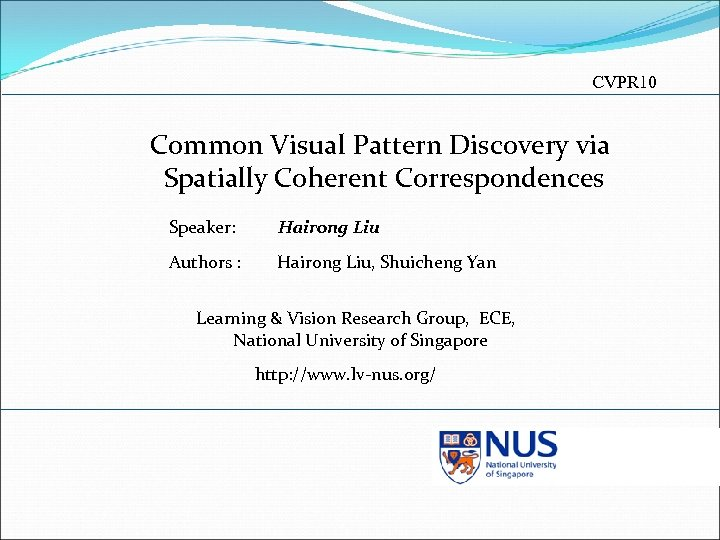CVPR 10 Common Visual Pattern Discovery via Spatially Coherent Correspondences Speaker: Hairong Liu Authors : Hairong Liu, Shuicheng Yan Learning & Vision Research Group, ECE, National University of Singapore http: //www. lv-nus. org/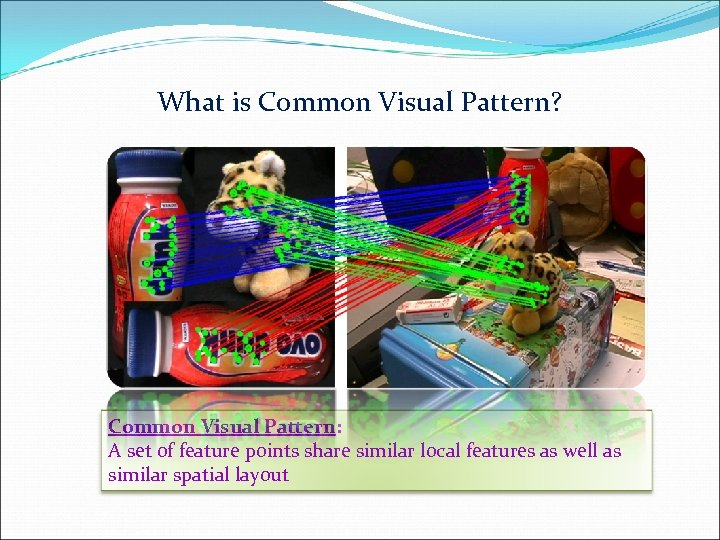What is Common Visual Pattern? Common Visual Pattern: A set of feature points share similar local features as well as similar spatial layout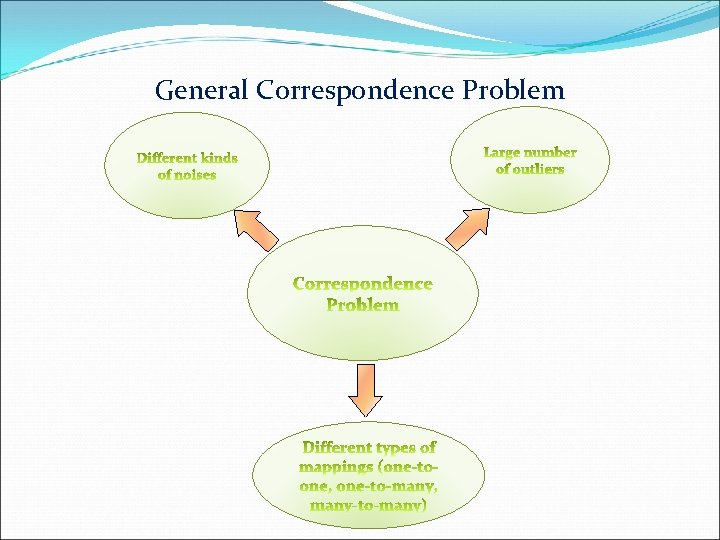General Correspondence Problem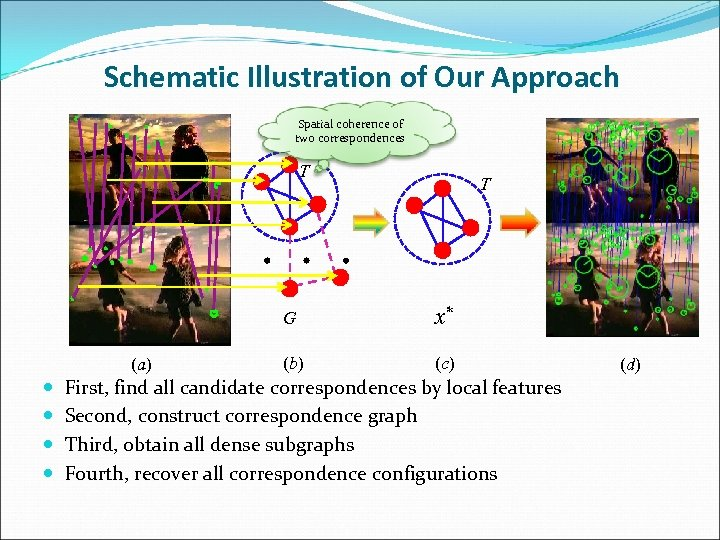Schematic Illustration of Our Approach Spatial coherence of two correspondences T T G (a) x* (b) (c) First, find all candidate correspondences by local features Second, construct correspondence graph Third, obtain all dense subgraphs Fourth, recover all correspondence configurations (d)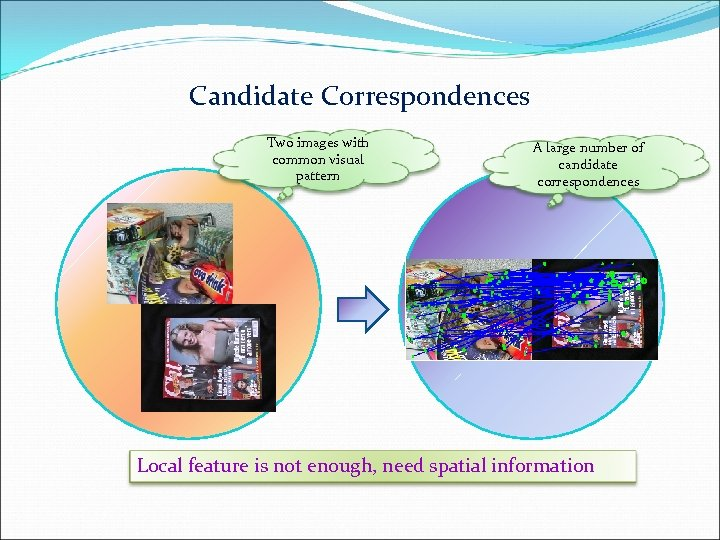Candidate Correspondences Two images with common visual pattern A large number of candidate correspondences Local feature is not enough, need spatial information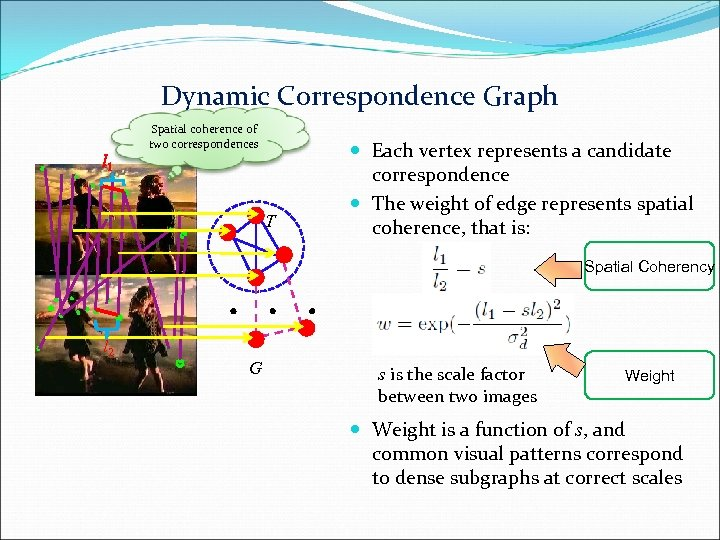Dynamic Correspondence Graph Spatial coherence of two correspondences l 1 T Each vertex represents a candidate correspondence The weight of edge represents spatial coherence, that is: Spatial Coherency l 2 G s is the scale factor between two images Weight is a function of s, and common visual patterns correspond to dense subgraphs at correct scales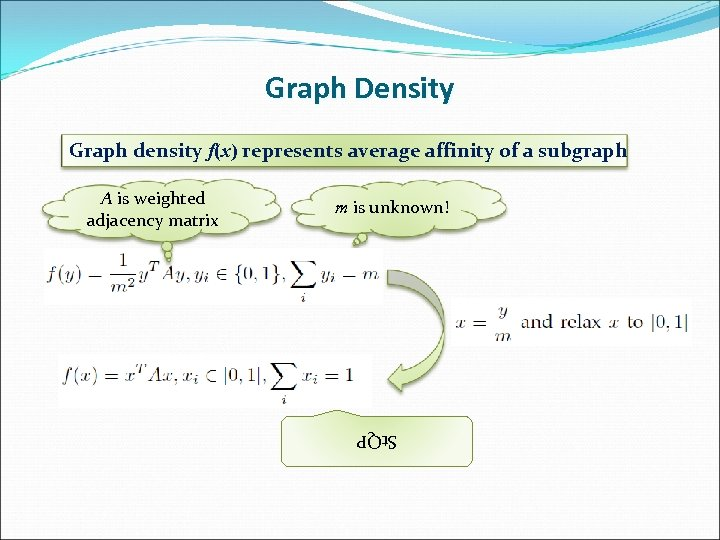Graph Density Graph density f(x) represents average affinity of a subgraph m is unknown! St. QP A is weighted adjacency matrix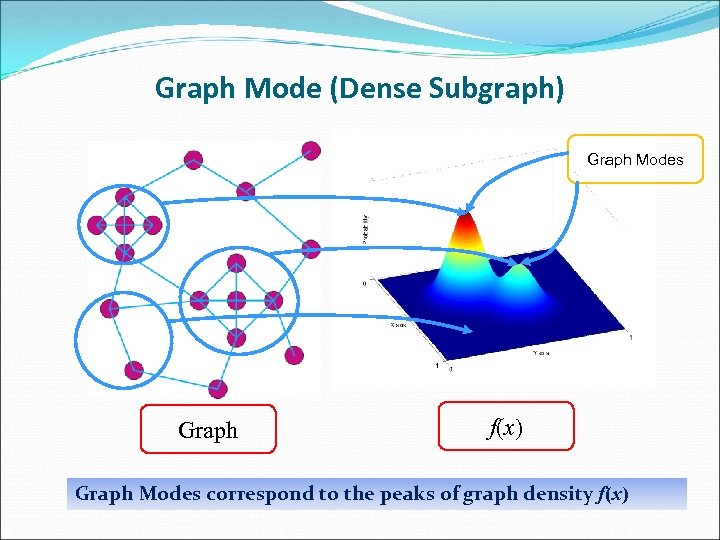Graph Mode (Dense Subgraph) Graph Modes Graph f(x) Graph Modes correspond to the peaks of graph density f(x)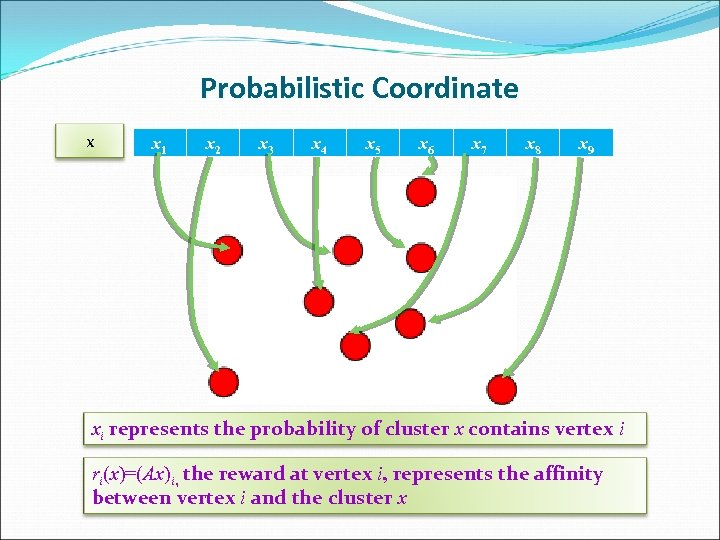Probabilistic Coordinate x x 1 x 2 x 3 x 4 x 5 x 6 x 7 x 8 x 9 xi represents the probability of cluster x contains vertex i ri(x)=(Ax)i, the reward at vertex i, represents the affinity between vertex i and the cluster x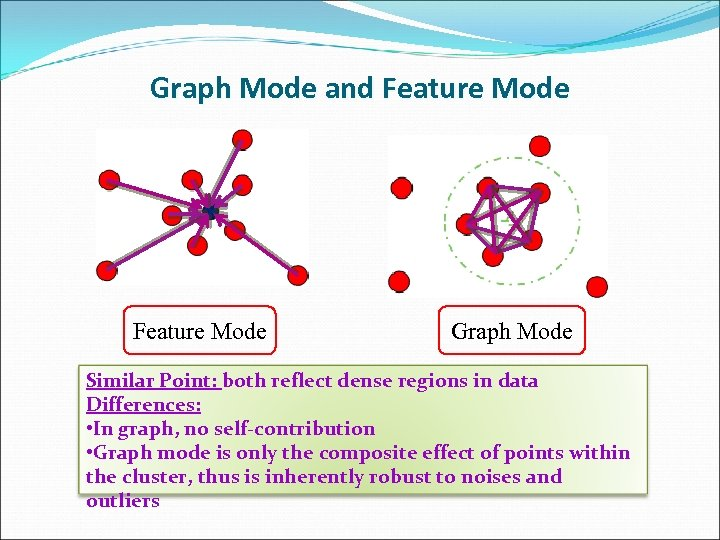Graph Mode and Feature Mode Graph Mode Similar Point: both reflect dense regions in data Differences: • In graph, no self-contribution • Graph mode is only the composite effect of points within the cluster, thus is inherently robust to noises and outliers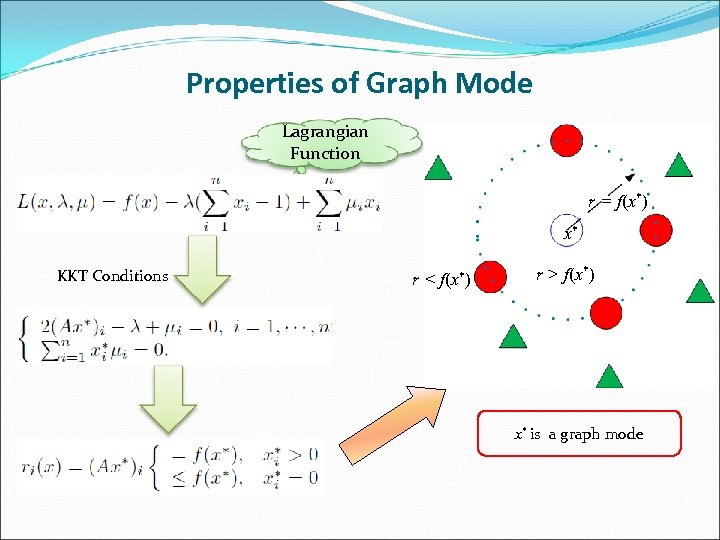Properties of Graph Mode Lagrangian Function r = f(x*) x* KKT Conditions r < f(x*) r > f(x*) x* is a graph mode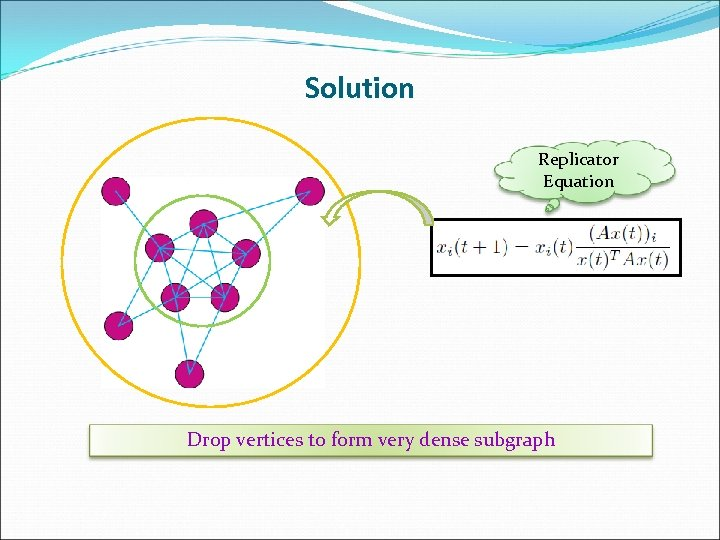Solution Replicator Equation Drop vertices to form very dense subgraph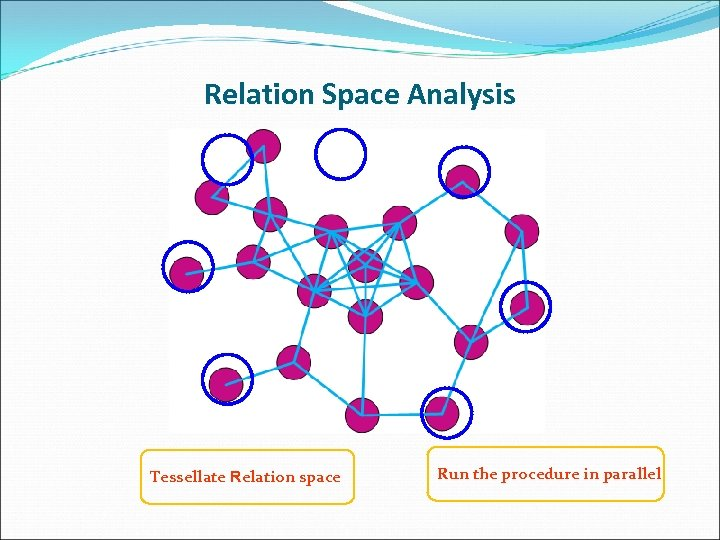Relation Space Analysis Tessellate Relation space Run the procedure in parallel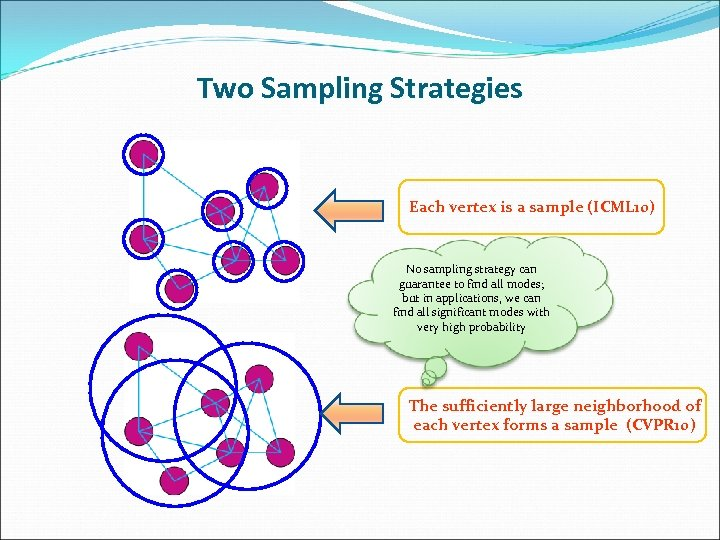Two Sampling Strategies Each vertex is a sample (ICML 10) No sampling strategy can guarantee to find all modes; but in applications, we can find all significant modes with very high probability The sufficiently large neighborhood of each vertex forms a sample (CVPR 10)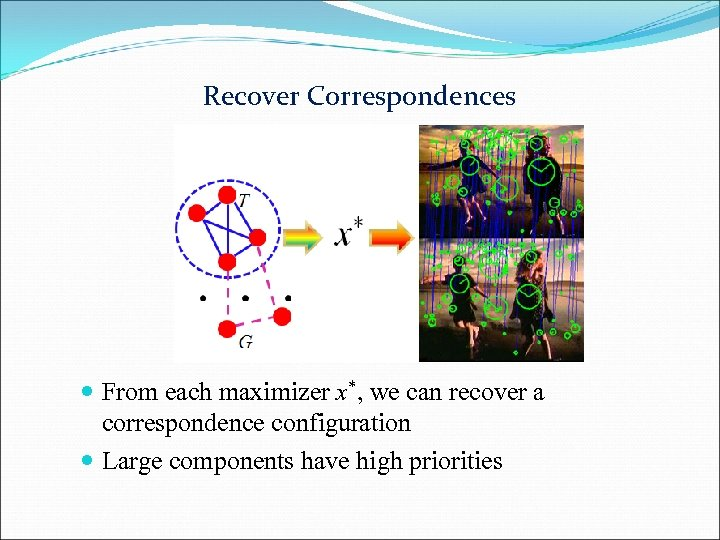Recover Correspondences From each maximizer x*, we can recover a correspondence configuration Large components have high priorities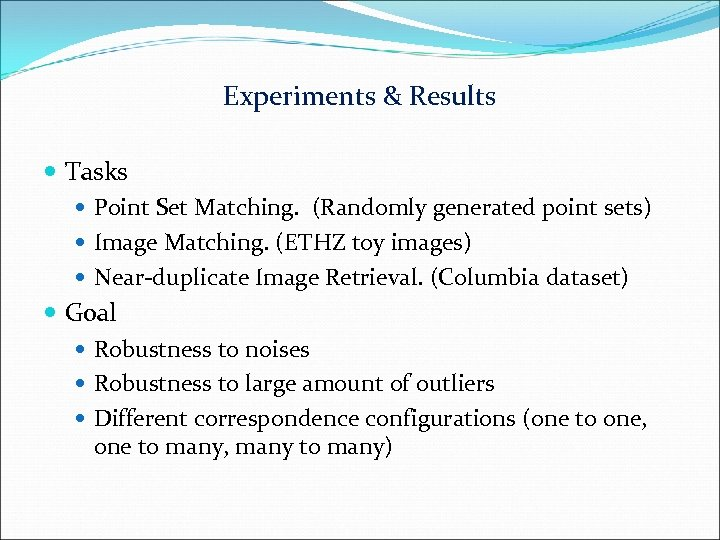Experiments & Results Tasks Point Set Matching. (Randomly generated point sets) Image Matching. (ETHZ toy images) Near-duplicate Image Retrieval. (Columbia dataset) Goal Robustness to noises Robustness to large amount of outliers Different correspondence configurations (one to one, one to many, many to many)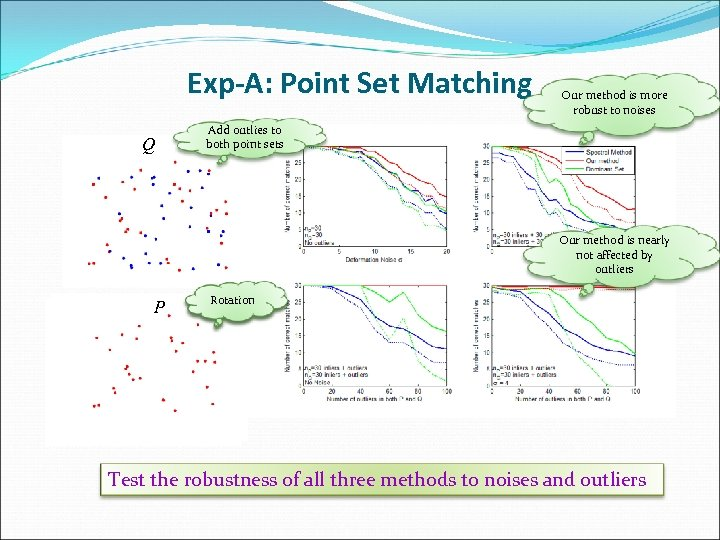Exp-A: Point Set Matching Q Our method is more robust to noises Add outlies to both point sets Our method is nearly not affected by outliers P Add Rotation noises Test the robustness of all three methods to noises and outliers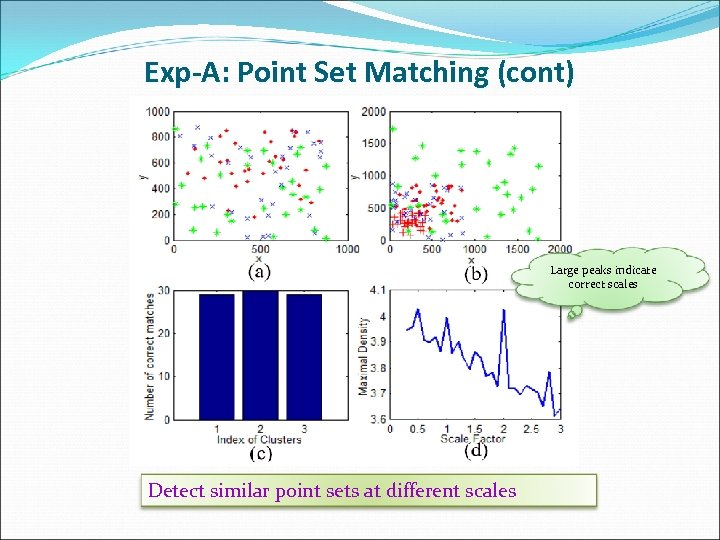Exp-A: Point Set Matching (cont) Large peaks indicate correct scales Detect similar point sets at different scales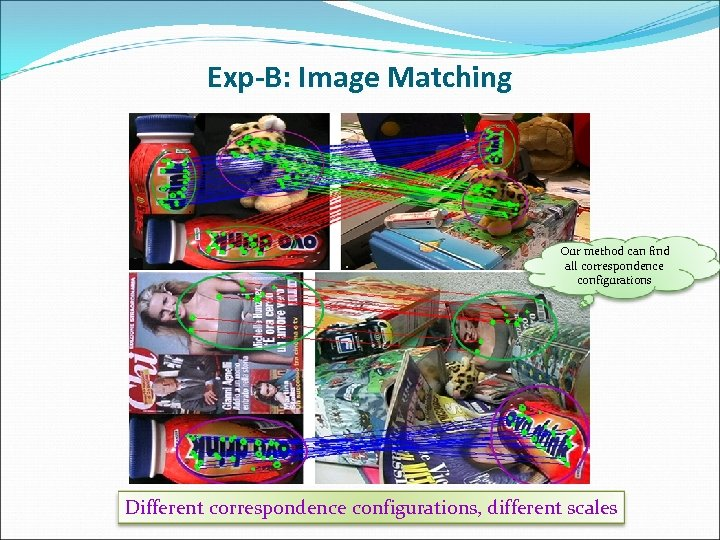Exp-B: Image Matching Our method can find all correspondence configurations Different correspondence configurations, different scales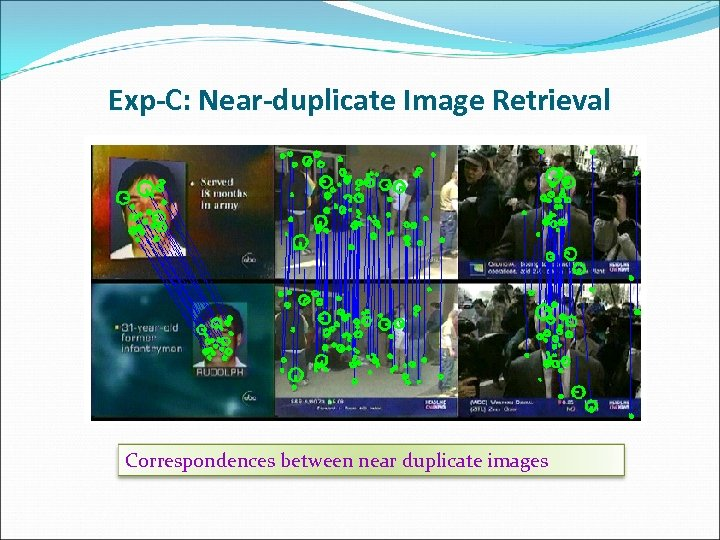Exp-C: Near-duplicate Image Retrieval Correspondences between near duplicate images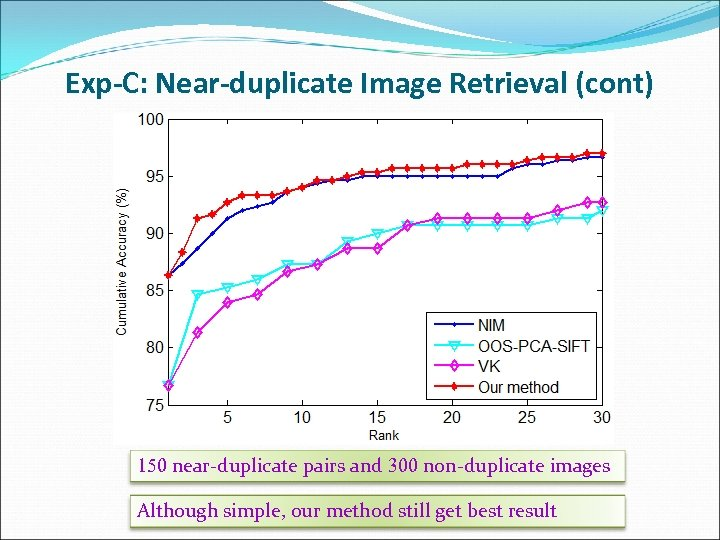Exp-C: Near-duplicate Image Retrieval (cont) 150 near-duplicate pairs and 300 non-duplicate images Although simple, our method still get best result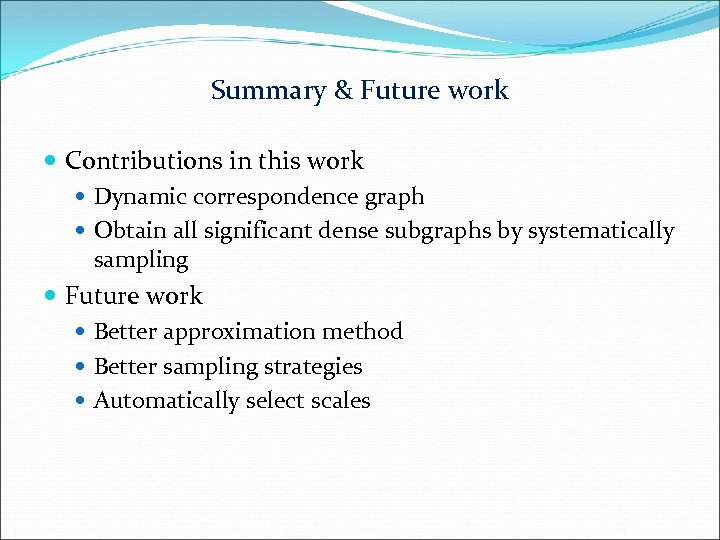Summary & Future work Contributions in this work Dynamic correspondence graph Obtain all significant dense subgraphs by systematically sampling Future work Better approximation method Better sampling strategies Automatically select scales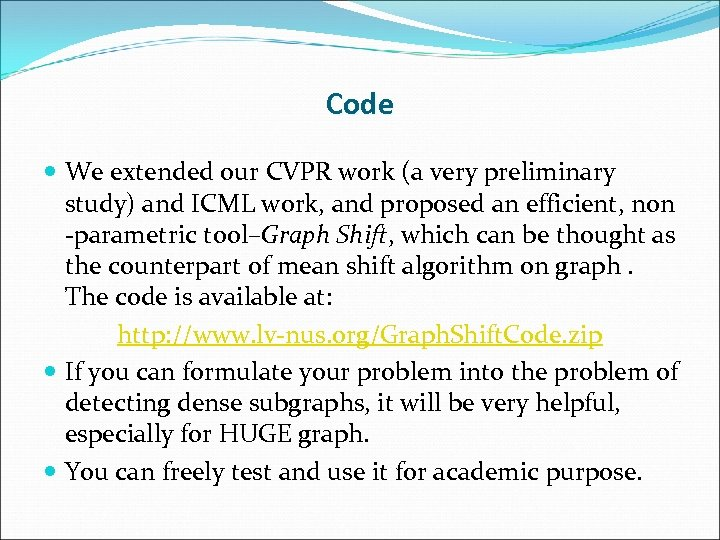Code We extended our CVPR work (a very preliminary study) and ICML work, and proposed an efficient, non -parametric tool–Graph Shift, which can be thought as the counterpart of mean shift algorithm on graph. The code is available at: http: //www. lv-nus. org/Graph. Shift. Code. zip If you can formulate your problem into the problem of detecting dense subgraphs, it will be very helpful, especially for HUGE graph. You can freely test and use it for academic purpose.